# Normed Spaces

Definition 18.1.1 (Norm)   Let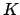be a field with valuation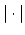and let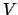be a vector space over. A real-valued functiononis called a if
1.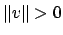for all nonzero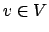(positivity).
2.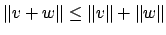for all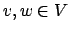(triangle inequality).
3.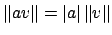for all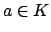and(homogeneity).

Note that setting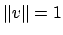for all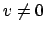does not define a norm unless the absolute value onis trivial, as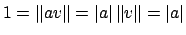. We assume for the rest of this section that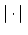is not trivial.

Definition 18.1.2 (Equivalent)   Two norms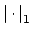and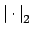on the same vector spaceare if there exists positive real numbers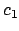and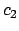such that for all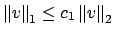and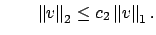Lemma 18.1.3   Suppose thatis a field that is complete with respect to a valuationand thatis a finite dimensionalvector space. Continue to assume, as mentioned above, thatis complete with respect to. Then any two norms onare equivalent.

Remark 18.1.4   As we shall see soon (see Theorem 19.1.8), the lemma is usually false if we do not assume thatis complete. For example, when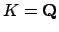and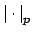is the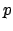-adic valuation, andis a number field, then there may be several extensions ofto inequivalent norms on.

If two norms are equivalent then the corresponding topologies onare equal, since very open ball foris contained in an open ball for, and conversely. (The converse is also true, since, as we will show, all norms onare equivalent.)

Proof. Let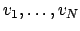be a basis for. Define the max norm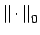by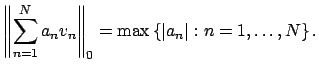It is enough to show that any normis equivalent to. We have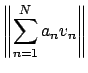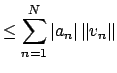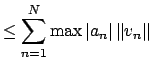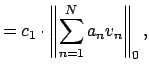where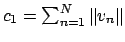.

To finish the proof, we show that there is a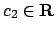such that for all,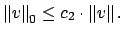We will only prove this in the case whenis not just merely complete with respect tobut also locally compact. This will be the case of primary interest to us. For a proof in the general case, see the original article by Cassels (page 53).

By what we have already shown, the function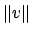is continuous in the-topology, so by local compactness it attains its lower bound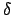on the unit circle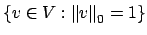. (Why is the unit circle compact? With respect to, the topology onis the same as that of a product of copies of. If the valuation is archimedean then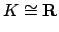or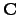with the standard topology and the unit circle is compact. If the valuation is non-archimedean, then we saw (see Remark 17.1.7) that ifis locally compact, then the valuation is discrete, in which case we showed that the unit disc is compact, hence the unit circle is also compact since it is closed.) Note that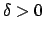by part 1 of Definition 18.1.1. Also, by definition of, for anythere existssuch that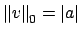(just take the max coefficient in our basis). Thus we can write anyas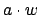whereand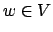with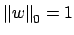. We then have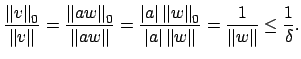Thus for all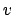we have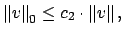where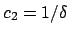, which proves the theorem.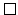William Stein 2004-05-06